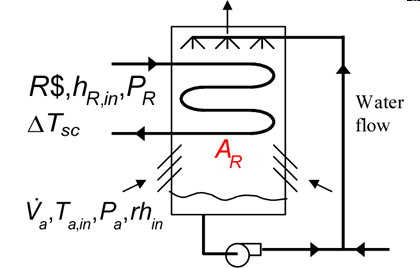Contents - index

EvaporativeCondenser1_CLThis procedure determines the requires surface area on the refrigerant side needed to condense a refrigerant (R\$) from its inlet state (which can be saturated or superheated) to a saturated or subcooled liquid.  Heat transfer coefficients for the air and refrigerant in the superheated, saturated and subcooled regimes are provided to the routine, along with the ratio of surface area on the air side to that on the refrigerant side.  The heat transfer coefficients can be determined using the functions in the Heat Transfer Library, as illustrated in the example.  The heat transfer enhancement resulting from the wetted surface is internally calculated using the method described by Mitchell and Braun (2013).  See Condenser1_CL and  Condenser3_CL for similar routines in which condensation occurs by heat transfer to dry air.  See EvaporativeCondenser2_CL for a similar procedure that determines the outlet states of the refrigerant and air given the surface area on the refrigerant side.

This library file can be used in the design stage of the evaporative condenser by inputting the design inlet conditions to determine the required area.

Inputs:

R\$:   name of the refrigerant

m_dot_R:  refrigerant flow rate (kg/s, lbm/hr)

h_R_in:  specific enthalpy of entering refrigerant (J/kg, kJ/kg, Btu/lbm)

DELTAT_sc:  number of degrees of subcooling (C, K, F, R)

P_R:  refrigerant pressure (Pa, kPa, bar, MPa, psia, atm)

V_dot:  volumetric flow rate of air (m^3/s, cfm)

T_air_in:  air inlet temperature  (C, K, F, R)

rh_in:  inlet air relative humidity (-)

P_air:  air pressure (Pa, kPa, bar, MPa, psia, atm)

h_air:  effective outside (air) heat transfer coefficient per unit refrigerant tube area (W/m^2-K, Btu/hr-ft^2-R)

A_air\A_R:  ratio of effective heat transfer area on the air side to the heat transfer area for the refrigerant*

h_R_SH:  heat transfer coefficient for refrigerant in the superheat regime  (W/m^2-K, Btu/hr-ft^2-R)

h_R_sat:  average heat transfer coefficient for refrigerant in the saturated regime  (W/m^2-K, Btu/hr-ft^2-R)

h_SC:  average heat transfer coefficient for refrigerant in the saturated regime  (W/m^2-K, Btu/hr-ft^2-R)

Outputs:

A_R:  required heat transfer area for the refrigerant (m^2, ft^2)

Q_dot:  overall heat transfer rate (W, kW, Btu/hr)

h_R_out: refrigerant outlet specific enthalpy (J/kg, kJ/kg, Btu/lbm)

T_air_out:  outlet temperature of the air  (C, K, F, R)

w_out: humidity ratio of exiting air (-)

f_sh fraction of the surface area in the superheat regime

f_sc fraction of the surface area in the subcooled regime

*A_air\A_R is the ratio of the product of the overall fin efficiency and total (finned and unfinned) area on the air side to the total surface area inside the refrigerant flow passages.  For unfinned surfaces, A_air\A_R is the ratio of the outer surface to inner surface areas.

Example:

\$UnitSystem Eng F psia

\$TabStops 0.2 4.5 in

\$VarInfo alpha units=1/ft

\$VarInfo A_finned units=ft^2

\$VarInfo A_unfinned units=ft^2

\$VarInfo A_tot Lower=10  Upper=5000  Guess=50 units=ft^2

\$VarInfo A_fr units=ft^2

\$Varinfo A_R units=ft^2

\$VarInfo D_h units=ft

\$VarInfo D_in units=ft

\$VarInfo D_o units=ft

\$VarInfo DELTAP_sc units=psia

\$VarInfo DELTAP_sh units=psia

\$VarInfo fin_pitch units=1/ft

\$VarInfo fin_thk units=ft

\$VarInfo h_R_in units=Btu/lb_m

\$VarInfo h_R_out units=Btu/lb_m

\$VarInfo h_air1 units=Btu/hr-ft^2-F

\$VarInfo h_cond units=Btu/hr-ft^2-F

\$VarInfo h_h_sc units=Btu/hr-ft^2-F

\$VarInfo h_h_sh units=Btu/hr-ft^2-F

\$VarInfo h_T_sc units=Btu/hr-ft^2-F

\$VarInfo h_T_sh units=Btu/hr-ft^2-F

\$VarInfo m_dot_air units=lb_m/hr

\$VarInfo m_dot_R_min units=lb_m/min

\$VarInfo P_R units=psia

\$VarInfo Q_dot units=Btu/hr

\$VarInfo T_a_out units=F

\$VarInfo T_R_in units=F

V_dot_air=10000 [cfm] "volumetric air flowrate"

T_air_in=80 [F] "air inlet temperature"

P_air=14.7 [psia] "air pressure"

rh_in=0.4 "air relative humidity"

R\$='R134a' "condensing refrigerant"

m_dot_R=800 [lbm/hr] "refrigerant flow rate"

m_dot_R_min=m_dot_R*convert(lbm/hr,lbm/min) "refrigerant flow rate in lbm/min"

T_R_sat=70 [F] "refrigerant saturation temperature"

P_R=p_sat(R\$,T=T_R_sat) "refrigerant saturation pressure"

Superheat=10 [DELTAF] "degrees of superheat at inlet"

T_R_in=T_R_sat+Superheat "inlet temperature"

h_R_in=enthalpy(R\$,T=T_R_in,x=1) "inlet specific enthalpy"

h_a=21.6 [Btu/hr-ft^2-R]   "heat transfer coefficient on air side - see below"

A_air\A_R=12.1  "ratio of area on air side to that on refrigerant side"

h_R_sh=36 [Btu/hr-ft^2-F] "heat transfer coefficient for refrigerant in superheat region"

h_R_sat=444 [Btu/hr-ft^2-R] "heat transfer coefficient for refrigerant in saturation region"

h_R_sc=31 [Btu/hr-ft^2-F]  "heat transfer coefficient for refrigerant in subcooled region"

DELTAT_sc=5 [DELTAF] "degrees of subcooling"

Call EvaporativeCondenser1_CL  (R\$, m_dot_R, h_R_in, DELTAT_sc, P_R, V_dot_air, T_air_in, rh_in, P_air, h_a, A_air\A_R, h_R_sh, h_R_sat, h_R_sc : A_R, Q_dot, h_R_out, T_a_out, w_out, f_sh, f_sc)

"!Calculation of the heat transfer coefficients using the Heat Transfer Library functions"

N_circuits=24 "number of parallel circuits"

A_fr=2*4 [ft^2] "frontal area"

W=4 [ft] "length of tubes"

N_tubes=A_R/(pi*W*D_in) "required number of tubes"

Call chx_geom_finned_tube('fc_tubes_s80-38T': D_o, fin_pitch, D_h, fin_thk, sigma, alpha, A_fin\A) "air side geometry"

"Determine ratio of area on air side to the refrigerant heat transfer area"

th = 0.9 [mm]*convert(mm,ft) "tube wall thickness"

D_in=D_o-2*th "inner tube diameter"

A_unfinned=pi*D_o*N_tubes*W*(1-fin_thk*fin_pitch) "unfinned area on air side"

A_tot=A_unfinned+A_finned "total finned and unfinned area on air side"

A_finned/A_tot=A_fin\A "ratio of finned to total area is provided by Compact HX library"

eta_o=0.97 "overall fin efficiency - See Heat Transfer, Nellis and Klein, section 8.5"

A_air\A_R1=eta_o*A_tot/(N_tubes*W*pi*D_in) "ratio of heat transfer area on air side to that on refrigerant side"

"Determine heat transfer coefficients for air and refrigerant "

m_dot_air=V_dot_air/volume(Air,T=T_air_in,P=P_air)*convert(lbm/min,lbm/hr) "air mass flow rate"

Call chx_h_finned_tube('fc_tubes_s80-38T', m_dot_air, A_fr, 'Air', T_air_in, P_air: h_air1) "compact hx correlation"

Call pipeflow('R134a',T_R_in, P_R, m_dot_R/N_circuits, D_in, N_tubes*W*f_sh, 0: h_T_sh, h_H_sh, DELTAP_sh, Nusselt_T_sh, f_R_sh, Re_R_sh) "superheat section"

T_w=65 [F] "estimated wall temperature"

Call cond_horizontaltube_avg(R\$, m_dot_R_min/N_Circuits, T_R_sat, T_w, D_in, 1, 0 : h_cond)  "saturated section"

Call pipeflow('R134a',T_R_sat-1 [F], P_R, m_dot_R/N_circuits, D_in, N_tubes*W*f_sc, 0: h_T_sc, h_H_sc, DELTAP_sc, Nusselt_T_sc, f_R_sc, Re_R_sc) "subcooled section"

{Solution:

A_R=65.59 [ft^2]

f_sc=0.1323

f_sh=0.1633

h_R_out=33.1 [Btu/lb_m]

Q_dot=64670 [Btu/hr]

T_a_out=65.31 [F]

w_out=0.01334

}